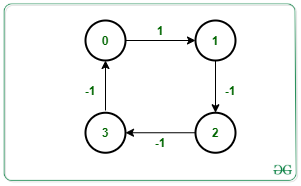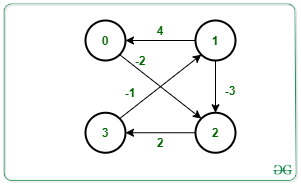# Detect a negative cycle in a Graph using Shortest Path Faster Algorithm

• Difficulty Level : Medium
• Last Updated : 10 Aug, 2021

Given a graph G consisting of nodes valued [0, N – 1], a source S, and an array Edges[] of type {u, v, w} that denotes that there is a directed edge between node u and v with weight w, the task is to check if there exists a negative cycle from the given source. If found to be true, then print “Yes”. Otherwise, print “No”.

A negative cycle is a cycle in which the sum of all its weight in that cycle is negative.

Examples:

Input: N = 4, M = 4, Edges[][] = {{0, 1, 1}, {1, 2, -1}, {2, 3, -1}, {3, 0, -1}}, S = 0
Output: Yes
Explanation:Starting from the source node 0, the graph contains cycle as 0 -> 1 -> 2 -> 3 -> 0.
The sum of weight in the above path is 1 – 1 – 1 – 1 = -2.
Therefore, the graph contains a negative cycle.

Input: N = 4, M = 5, Edges[][] = {{0, 2, -2}, {1, 0, 4}, {1, 2, -3}, {2, 3}, {3, 1}}, W[] = {-2, 4, -3, 2, -1}, S = 1
Output: Yes
Explanation:Starting from the source node 1, the graph contains cycle as 1 -> 2 -> 3 -> 1.
The sum of weight in the above path is -3 + 2 – 1 = -2.
Therefore, the graph contains a negative cycle.

Approach: The idea is to use the Shortest Path Faster Algorithm(SPFA) to find if a negative cycle is present and reachable from the source vertex in a graph. Follow the below steps to solve the problem:

• Initialize the arrays dis[] with large value, vis[] with false and cnt[] to store the count about how many times a vertex has been relaxed.
• Traverse the graph using the SPFA algorithm.
• Increment the count for each vertex whenever the vertex is relaxed.

The term relaxation means updating the cost of all vertices connected to a vertex v if those costs would be improved by including the path via vertex v.

• Stop the algorithm and print “Yes” as soon as some vertex got relaxed for the Nth time as there are only N vertices i.e., from 0 to N – 1.
• Otherwise, print “No”.

Below is the implementation of the above approach:

## C++

 `// C++ program for the above approach` `#include ` `using` `namespace` `std;`   `bool` `sfpa(``int` `V, ``int` `src, ``int` `Edges[],` `          ``int` `M)` `{` `    ``// Stores the adjacency list of` `    ``// the given graph` `    ``vector > g[V];`   `    ``// Create Adjacency List` `    ``for` `(``int` `i = 0; i < M; i++) {`   `        ``int` `u = Edges[i];` `        ``int` `v = Edges[i];` `        ``int` `w = Edges[i];`   `        ``g[u].push_back({ v, w });` `    ``}`   `    ``// Stores the distance of all` `    ``// reachable vertex from source` `    ``vector<``int``> dist(V, INT_MAX);`   `    ``// Check if vertex is present` `    ``// in queue or not` `    ``vector<``bool``> inQueue(V, ``false``);`   `    ``// Counts the relaxation for` `    ``// each vertex` `    ``vector<``int``> cnt(V, 0);`   `    ``// Distance from src to src is 0` `    ``dist[src] = 0;`   `    ``// Create a queue` `    ``queue<``int``> q;`   `    ``// Push source in the queue` `    ``q.push(src);`   `    ``// Mark source as visited` `    ``inQueue[src] = ``true``;`   `    ``while` `(!q.empty()) {`   `        ``// Front vertex of Queue` `        ``int` `u = q.front();` `        ``q.pop();`   `        ``inQueue[u] = ``false``;`   `        ``// Relaxing all edges of` `        ``// vertex from the Queue` `        ``for` `(pair<``int``, ``int``> x : g[u]) {`   `            ``int` `v = x.first;` `            ``int` `cost = x.second;`   `            ``// Update the dist[v] to` `            ``// minimum distance` `            ``if` `(dist[v] > dist[u] + cost) {`   `                ``dist[v] = dist[u] + cost;`   `                ``// If vertex v is in Queue` `                ``if` `(!inQueue[v]) {` `                    ``q.push(v);` `                    ``inQueue[v] = ``true``;` `                    ``cnt[v]++;`   `                    ``// Negative cycle` `                    ``if` `(cnt[v] >= V)` `                        ``return` `true``;` `                ``}` `            ``}` `        ``}` `    ``}`   `    ``// No cycle found` `    ``return` `false``;` `}`   `// Driver Code` `int` `main()` `{` `    ``// Number of vertices` `    ``int` `N = 4;`   `    ``// Given source node src` `    ``int` `src = 0;`   `    ``// Number of Edges` `    ``int` `M = 4;`   `    ``// Given Edges with weight` `    ``int` `Edges[] = { { 0, 1, 1 },` `                       ``{ 1, 2, -1 },` `                       ``{ 2, 3, -1 },` `                       ``{ 3, 0, -1 } };`   `    ``// If cycle is present` `    ``if` `(sfpa(N, src, Edges, M) == ``true``)` `        ``cout << ``"Yes"` `<< endl;` `    ``else` `        ``cout << ``"No"` `<< endl;`   `    ``return` `0;` `}`

## Java

 `// Java program for ` `// the above approach` `import` `java.util.*;` `class` `GFG{` `    `  `static` `class` `pair` `{ ` `  ``int` `first, second; ` `  ``public` `pair(``int` `first, ` `              ``int` `second)  ` `  ``{ ` `    ``this``.first = first; ` `    ``this``.second = second; ` `  ``}    ` `} ` `  `  `static` `boolean` `sfpa(``int` `V, ``int` `src, ` `                    ``int` `Edges[][], ``int` `M)` `{` `  ``// Stores the adjacency list of` `  ``// the given graph` `  ``Vector []g = ``new` `Vector[V];` `  ``for` `(``int` `i = ``0``; i < V; i++) ` `  ``{` `    ``g[i] = ``new` `Vector();` `  ``}`   `  ``// Create Adjacency List` `  ``for` `(``int` `i = ``0``; i < M; i++) ` `  ``{` `    ``int` `u = Edges[i][``0``];` `    ``int` `v = Edges[i][``1``];` `    ``int` `w = Edges[i][``2``];` `    ``g[u].add(``new` `pair(v, w));` `  ``}`   `  ``// Stores the distance of all` `  ``// reachable vertex from source` `  ``int` `[]dist = ``new` `int``[V];` `  ``Arrays.fill(dist, Integer.MAX_VALUE);`   `  ``// Check if vertex is present` `  ``// in queue or not` `  ``boolean` `[]inQueue = ``new` `boolean``[V];`   `  ``// Counts the relaxation for` `  ``// each vertex` `  ``int` `[]cnt = ``new` `int``[V];`   `  ``// Distance from src ` `  ``// to src is 0` `  ``dist[src] = ``0``;`   `  ``// Create a queue` `  ``Queue q = ``new` `LinkedList<>();`   `  ``// Push source in the queue` `  ``q.add(src);`   `  ``// Mark source as visited` `  ``inQueue[src] = ``true``;`   `  ``while` `(!q.isEmpty()) ` `  ``{` `    ``// Front vertex of Queue` `    ``int` `u = q.peek();` `    ``q.remove();`   `    ``inQueue[u] = ``false``;`   `    ``// Relaxing all edges of` `    ``// vertex from the Queue` `    ``for` `(pair x : g[u]) ` `    ``{` `      ``int` `v = x.first;` `      ``int` `cost = x.second;`   `      ``// Update the dist[v] to` `      ``// minimum distance` `      ``if` `(dist[v] > dist[u] + cost) ` `      ``{` `        ``dist[v] = dist[u] + cost;`   `        ``// If vertex v is in Queue` `        ``if` `(!inQueue[v]) ` `        ``{` `          ``q.add(v);` `          ``inQueue[v] = ``true``;` `          ``cnt[v]++;`   `          ``// Negative cycle` `          ``if` `(cnt[v] >= V)` `            ``return` `true``;` `        ``}` `      ``}` `    ``}` `  ``}`   `  ``// No cycle found` `  ``return` `false``;` `}`   `// Driver Code` `public` `static` `void` `main(String[] args)` `{` `  ``// Number of vertices` `  ``int` `N = ``4``;`   `  ``// Given source node src` `  ``int` `src = ``0``;`   `  ``// Number of Edges` `  ``int` `M = ``4``;`   `  ``// Given Edges with weight` `  ``int` `Edges[][] = {{``0``, ``1``, ``1``},` `                   ``{``1``, ``2``, -``1``},` `                   ``{``2``, ``3``, -``1``},` `                   ``{``3``, ``0``, -``1``}};`   `  ``// If cycle is present` `  ``if` `(sfpa(N, src, Edges, M) == ``true``)` `    ``System.out.print(``"Yes"` `+ ``"\n"``);` `  ``else` `    ``System.out.print(``"No"` `+ ``"\n"``);` `}` `}`   `// This code is contributed by 29AjayKumar`

## Python3

 `# Python3 program for the above approach` `import` `sys`   `def` `sfpa(V, src, Edges, M):` `    `  `    ``# Stores the adjacency list of` `    ``# the given graph` `    ``g ``=` `[[] ``for` `i ``in` `range``(V)]`   `    ``# Create Adjacency List` `    ``for` `i ``in` `range``(M):` `        ``u ``=` `Edges[i][``0``]` `        ``v ``=` `Edges[i][``1``]` `        ``w ``=` `Edges[i][``2``]`   `        ``g[u].append([v, w])`   `    ``# Stores the distance of all` `    ``# reachable vertex from source` `    ``dist ``=` `[sys.maxsize ``for` `i ``in` `range``(V)]`   `    ``# Check if vertex is present` `    ``# in queue or not` `    ``inQueue ``=` `[``False` `for` `i ``in` `range``(V)]`   `    ``# Counts the relaxation for` `    ``# each vertex` `    ``cnt ``=` `[``0` `for` `i ``in` `range``(V)]`   `    ``# Distance from src to src is 0` `    ``dist[src] ``=` `0`   `    ``# Create a queue` `    ``q ``=` `[]`   `    ``# Push source in the queue` `    ``q.append(src)`   `    ``# Mark source as visited` `    ``inQueue[src] ``=` `True`   `    ``while` `(``len``(q)):` `        `  `        ``# Front vertex of Queue` `        ``u ``=` `q[``0``]` `        ``q.remove(q[``0``])`   `        ``inQueue[u] ``=` `False`   `        ``# Relaxing all edges of` `        ``# vertex from the Queue` `        ``for` `x ``in` `g[u]:` `            ``v ``=` `x[``0``]` `            ``cost ``=` `x[``1``]`   `            ``# Update the dist[v] to` `            ``# minimum distance` `            ``if` `(dist[v] > dist[u] ``+` `cost):` `                ``dist[v] ``=` `dist[u] ``+` `cost`   `                ``# If vertex v is in Queue` `                ``if` `(inQueue[v] ``=``=` `False``):` `                    ``q.append(v)` `                    ``inQueue[v] ``=` `True` `                    ``cnt[v] ``+``=` `1`   `                    ``# Negative cycle` `                    ``if` `(cnt[v] >``=` `V):` `                        ``return` `True`   `    ``# No cycle found` `    ``return` `False`   `# Driver Code` `if` `__name__ ``=``=` `'__main__'``:` `    `  `    ``# Number of vertices` `    ``N ``=` `4`   `    ``# Given source node src` `    ``src ``=` `0`   `    ``# Number of Edges` `    ``M ``=` `4`   `    ``# Given Edges with weight` `    ``Edges ``=` `[ [ ``0``, ``1``, ``1` `],` `              ``[ ``1``, ``2``, ``-``1` `],` `              ``[ ``2``, ``3``, ``-``1` `],` `              ``[ ``3``, ``0``, ``-``1` `] ]`   `    ``# If cycle is present` `    ``if` `(sfpa(N, src, Edges, M) ``=``=` `True``):` `        ``print``(``"Yes"``)` `    ``else``:` `        ``print``(``"No"``)`   `# This code is contributed by SURENDRA_GANGWAR`

## C#

 `// C# program for ` `// the above approach` `using` `System;` `using` `System.Collections.Generic;` `class` `GFG{` `    `  `class` `pair` `{ ` `  ``public` `int` `first, second; ` `  ``public` `pair(``int` `first, ` `              ``int` `second)  ` `  ``{ ` `    ``this``.first = first; ` `    ``this``.second = second; ` `  ``}    ` `} ` `  `  `static` `bool` `sfpa(``int` `V, ``int` `src, ` `                 ``int` `[,]Edges, ``int` `M)` `{` `  ``// Stores the adjacency list of` `  ``// the given graph` `  ``List []g = ``new` `List[V];` `  ``for` `(``int` `i = 0; i < V; i++) ` `  ``{` `    ``g[i] = ``new` `List();` `  ``}`   `  ``// Create Adjacency List` `  ``for` `(``int` `i = 0; i < M; i++) ` `  ``{` `    ``int` `u = Edges[i, 0];` `    ``int` `v = Edges[i, 1];` `    ``int` `w = Edges[i, 2];` `    ``g[u].Add(``new` `pair(v, w));` `  ``}`   `  ``// Stores the distance of all` `  ``// reachable vertex from source` `  ``int` `[]dist = ``new` `int``[V];` `  ``for` `(``int` `i = 0; i < V; i++) ` `    ``dist[i] = ``int``.MaxValue;`   `  ``// Check if vertex is present` `  ``// in queue or not` `  ``bool` `[]inQueue = ``new` `bool``[V];`   `  ``// Counts the relaxation for` `  ``// each vertex` `  ``int` `[]cnt = ``new` `int``[V];`   `  ``// Distance from src ` `  ``// to src is 0` `  ``dist[src] = 0;`   `  ``// Create a queue` `  ``Queue<``int``> q = ``new` `Queue<``int``>();`   `  ``// Push source in the queue` `  ``q.Enqueue(src);`   `  ``// Mark source as visited` `  ``inQueue[src] = ``true``;`   `  ``while` `(q.Count != 0) ` `  ``{` `    ``// Front vertex of Queue` `    ``int` `u = q.Peek();` `    ``q.Dequeue();`   `    ``inQueue[u] = ``false``;`   `    ``// Relaxing all edges of` `    ``// vertex from the Queue` `    ``foreach` `(pair x ``in` `g[u]) ` `    ``{` `      ``int` `v = x.first;` `      ``int` `cost = x.second;`   `      ``// Update the dist[v] to` `      ``// minimum distance` `      ``if` `(dist[v] > dist[u] + cost) ` `      ``{` `        ``dist[v] = dist[u] + cost;`   `        ``// If vertex v is in Queue` `        ``if` `(!inQueue[v]) ` `        ``{` `          ``q.Enqueue(v);` `          ``inQueue[v] = ``true``;` `          ``cnt[v]++;`   `          ``// Negative cycle` `          ``if` `(cnt[v] >= V)` `            ``return` `true``;` `        ``}` `      ``}` `    ``}` `  ``}`   `  ``// No cycle found` `  ``return` `false``;` `}`   `// Driver Code` `public` `static` `void` `Main(String[] args)` `{` `  ``// Number of vertices` `  ``int` `N = 4;`   `  ``// Given source node src` `  ``int` `src = 0;`   `  ``// Number of Edges` `  ``int` `M = 4;`   `  ``// Given Edges with weight` `  ``int` `[,]Edges = {{0, 1, 1},` `                  ``{1, 2, -1},` `                  ``{2, 3, -1},` `                  ``{3, 0, -1}};`   `  ``// If cycle is present` `  ``if` `(sfpa(N, src, Edges, M) == ``true``)` `    ``Console.Write(``"Yes"` `+ ``"\n"``);` `  ``else` `    ``Console.Write(``"No"` `+ ``"\n"``);` `}` `}`   `// This code is contributed by 29AjayKumar`

## Javascript

 ``

Output:

`Yes`

Time Complexity: O(N*M), where N is the number of vertices and M is the number of edges.
Auxiliary Space: O(N + M)

My Personal Notes arrow_drop_up
Recommended Articles
Page :